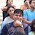## Pages

### REASONING Trick of TIME MIRROR IMAGE WATER IMAGE

REASONING Trick of TIME MIRROR IMAGE WATER IMAGE
CLOCK TIME MIRROR IMAGE WATER IMAGE are mandatory part covers 2-3 questions in Reasoning section of SSC or other competitive exams. Such questions are very easy to solve within 2-3 seconds by using FASTEST MEMORY TABLE TRICKS. REASONING Trick of TIME MIRROR IMAGE WATER IMAGE SIMPLE TRICKS provides accurate solution and helps in getting maximum score in SSC IBPS SBI or other competitive exam. This SIMPLE TRICKS provide memory tricks to remember TIME TABLE for water and mirror images.

### REASONING Trick of TIME MIRROR IMAGE WATER IMAGE

Aspirants are recommended to use SIMPLE TRICKS to solve CLOCK TIME MIRROR IMAGE WATER IMAGE in Reasoning section to get maximum score in exam.

RULES FOR SIMPLE TRICKS - REASONING Trick of TIME MIRROR IMAGE WATER IMAGE

1.      In one revolution 360o, an Hour hand makes 30o, a Minute hand makes 6o and Second hand makes 6o.
2.      For Mirror image of Clock Time, the given time shall be subtracted from 12:00 or 11:60.
3.      For Water image of Clock Time, the given time shall be subtracted from 18:30
-------------------------------
Question 1:
What will be mirror image of Clock Time 3: 40
Solution: By Using Simple Tricks (Rule 2)
Do subtraction                                    11: 60
3: 40
-----------
8 : 20
-----------
Question 2:
What will be Water image of Clock Time 3: 20
Solution: By Using Simple Tricks (Rule 3)
Do subtraction                                    18: 30
3: 20
-----------
15: 10
-----------
Do the practices for different coded blood relationship questions from previous papers set to achieve cut-off score in SSC IBPS or other competitive exams.

1.Thank you ruby :)

2.Thanks... This made solving easier and quicker

3.4.5.How to find the water image of the given minutes are greater than 30? Then how to subtract. I MEAN.. if the given timing is 3:40 and we need to subtract it from 18:30 then what to do... should we subtrat it by taking a carry??

1.YEAH YOU WILL SUBTRACT from 17:90.
17:90 (1hr=60min,so 18-1=17hr, 30+60=90min)
-03:40
_______
14:50 in 24 hrs
-12:00
_______
2:50 in 12 hrs.

2.6.The answer for clock images beyond 18:30 that is let's say for 20:22 doesn't match.

7.Sir 3:30 ki water image kya bnegi show me the figure plz..

1.2.3.8.why do we substract the time from 18:30, to find the water image.

9.12 bje ki water image is hisab se 6 :30 hoga pr 12 bje dono hand ak sath hote he this is not the case with 6:30.

10.11.1.5:00 100% correct

2.5:00 100% correct.

12.Koi concept ke according solve kre

4:25 and 2:10 ka water Image time kya hoga

13.what is reason to subtract the given time form 18hr:30min to obtained time image in water

14.क्या आप बता सकते है कि हम पानी में छवि को प्राप्त करने के लिए दिए गए समय को हमेशा 18:30 में से ही क्यों घटाते हैं?